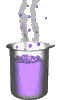Science class online
Chemistry and physics lessons

# Chemistry

Free online chemistry lesson for elementary school, middle school and high school.Science class Chemistry Electricity Optics Mechanics Mechanics lessons Water Water on Earth Changes of state in the nature: the water cycle Water in human body Test for water Properties of water in different states States of matter States of matter and its changes Boiling water Water: freezing and melting Changes of state: mass and volume Molecules in different states of matter Mixtures and solutions Heterogeneous mixtures Homogeneous mixtures Decantation Centrifugation Filtration Vaporization of water Distillation Chromatography Dissolving a solid in water Conservation of mass on dissolving Miscibility in water Mass and volume Volume and its units Measuring volume with a graduated cylinder Mass and its units Measuring the mass of a liquid Mass of a liter of waterVolumetric mass density Air and atmosphere Earth's atmosphere protect us Pressure Atoms and molecules Molecules Molecules in mixtures and pure substances Molecules and states of matter Atoms Composition of molecules Constituents of the atoms The electrical neutrality of the atom Combustions Basics of combustion The combustion of carbon The combustion of butane Atoms and chemical reactions Chemical reactions Chemical equations Law of conservation of mass Chemical synthesis Metals Corrosion of metals Electrical conductivity of solid materials Electrons and free electrons Electric current in metals Ions The conductivity of aqueous solutions Aqueous solutions and ions The direction of movement of ions Formation of ions Tests for ions Acidic and basic solutions pH of aqueous solutions Ions in acidic and basic solutions Dilution of acids and bases Composition of hydrochloric acid Chemical reaction between iron and hydrochloric acid Electrochemical cells and chemical energy Chemical reaction beteween a copper sulphate solution and zinc Copper sulfate and zinc battery Basics of electrochemical cell

 _____________________________________________________   _____________________________________________________

## Atoms and chemical reactions

### Chemical equation

 1) What is a chemical reaction ? The reaction equation is another way (more accurate than a simple word equation) to describe a chemical transformation. The reaction equation is written starting from the simple word equation: - The names of products and reactants are replaced by their chemical formula. - The law of conservation of matter must be respected. The law of conservation of matter: The number of atoms of each type is the same before and after the reaction. ______________________________________ ______________________________________ 2) Chemical equation for combustion of carbon Simple word equation: Carbon + dioxygen   →   carbone dioxide Chemical equation: Step 1: names are replaced by their chemical formula C + O2   →   CO2 Step 2: verification of law of conservation of matter Total number of atoms before          Total number of atoms after chemical transformation:                    chemical transformation: * Carbon atoms: 1                            *  Carbon atoms: 1       * Oxygen atoms:   2                          *  Oxygen atoms: 2   Law of conservation of matter is then respected: it is said that the equation is balanced This equation is read: A carbon atom and an oxygen molecule react together to give a molecule of oxygen. 3) Chemical equation for combustion of méthan Simple word equation: methane + dioxygen   →   water + carbon dioxid Chemical equation (temporary)  CH4 + O2    →   H2O + CO2 Total number of atoms before                  Total number of atoms after chemical transformation:                          chemical transformation: * Carbon atoms: 1                                     *  Carbon atoms: 1             * Oxygen atoms:  2                                    *   Oxygen atoms:  1 + 2 = 3 * Hydrogen atoms:  4                                *   Hydrogen atoms:  2 This equation is not balanced since the number of oxygen atoms and hydrogen atoms are not the same in the reactants and products. It should be added a coefficient before some formulas to modify the number of molecules:  CH4 + 2 O2  →  2 H2O + CO2 Total number of atoms before                  Total number of atoms after chemical transformation:                          chemical transformation: * Carbon atoms: 1                                     *  Carbon atoms: 1             * Oxygen atoms:  2 x 2 = 4                       *   Oxygen atoms:  2 x 1 + 2 = 4 * Hydrogen atoms:  4                                *   Hydrogen atoms:  2 x 2 = 4 The equation is then balanced The chemical reaction can be read as follow: A methane molecule and two dioxygen molecules give two molecules of water and one molecule of carbon dioxide. ______________________________________ ______________________________________
____________________________________

____________________________________